Uniformly integrable set of random variables

A set of random variables (cf. Random variable) having finite expectations such that integrated tails of their distribution functions are uniformly small. Let a set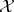consist of random variables defined on a common probability space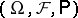. It is called uniformly integrable if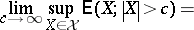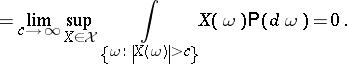Uniform integrability is a kind of compactness of sets of random variables or their distribution functions. It plays a key role in a variety of convergence problems. An example of this is the following theorem [a1].

Theorem 1.

Let a sequenceof random variables such that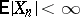,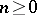, converge in probability to a random variable(cf. Convergence in probability). Then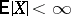and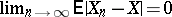if and only if the setis uniformly integrable.

In fact, the definition of uniform integrability is stated in terms of marginal distribution functions of random variables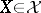and does not necessarily require that all these random variables are defined on the same probability space.

Each finite set of random variables having finite absolute expectations is uniformly integrable. This does not hold, in general, for infinite sets.

Theorem 2.

A setof random variables is uniformly integrable if and only if there exists a non-negative increasing convex functionsuch that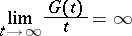and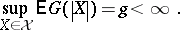The criterion above leads to a quantification of the notion of uniform integrability: The straightforward estimate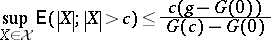represents a uniform upper bound of the integrated tails of all random variables belonging to a uniformly integrable set.